Technical Article

# Noise Figure Measurement Using the Y-Factor Method: Noise Source Uncertainty and Fixturing Losses

August 11, 2023 by Dr. Steve Arar

## The Y-factor method simplifies noise figure measurements by allowing the use of variable attenuators in place of a calibrated power meter. In addition to defining the Y factor, we will discuss noise source uncertainty and fixturing losses that can introduce errors in your noise figure measurements.

Modern automated noise figure (NF) instruments make it quite easy to perform noise figure measurements. However, they do not eliminate the need for a knowledgeable operator. If you don’t have a solid understanding of the error sources affecting your measurement, then automating that measurement will not make it better. As Dr. Thomas H. Lee stated in Planar Microwave Engineering, “Automated equipment merely lets you produce more wrong answers per unit time.”

### Y Factor is a Ratio

Figure 1 shows a simplified block diagram of the Y-factor method for NF measurement.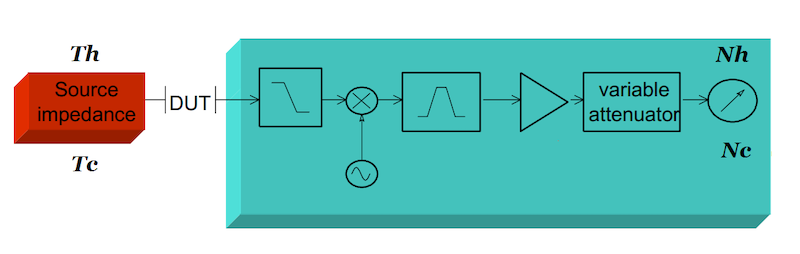##### Figure 1. Simplified block diagram for the Y-factor method of noise figure measurement. Image used courtesy of Agilent Technologies

The Y-factor method applies two different noise levels with known equivalent temperatures of Th (hot) and Tc (cold) to the device under test’s (DUT’s) input and measures the corresponding output noise powers Nh and Nc. The Y factor is defined as the ratio of Nh to Nc:

$$Y = \frac{N_h}{N_c}$$

##### Equation 1

The noise temperature, Te, of the DUT is found using the following equation:

$$T_e = \frac{T_h - YT_c}{Y-1}$$

##### Equation 2

A key concept here is that the Y-factor method requires the ratio of Nh to Nc, not their absolute values. This means that the measurement equipment can be built without relying on a calibrated power meter. Rather than using a calibrated power meter, NF instruments normally use a precision variable attenuator (Figure 1).

### Measuring the Y Factor

The basic operation is as follows:

1. Connect the cold source and set the attenuation factor to 1.
2. Measure the output noise level.
3. Connect the hot source.
4. Increase the attenuation factor gradually until the power meter shows the same reading as the cold source measurement.
5. The attenuation factor obtained from this measurement equals the ratio Nh/Nc. In other words, the attenuation factor actually gives us the Y factor.

This basic understanding of the operation of a noise figure analyzer can sometimes help us better understand the tips and tricks for making accurate measurements.

### Noise Source Uncertainty

To make noise figure measurements, a noise source with calibrated output noise level is required (example shown in Figure 2). The noise level of a noise source is characterized by its excessive noise ratio (ENR). The NF instrument uses the ENR values of the noise source to calculate the DUT noise figure.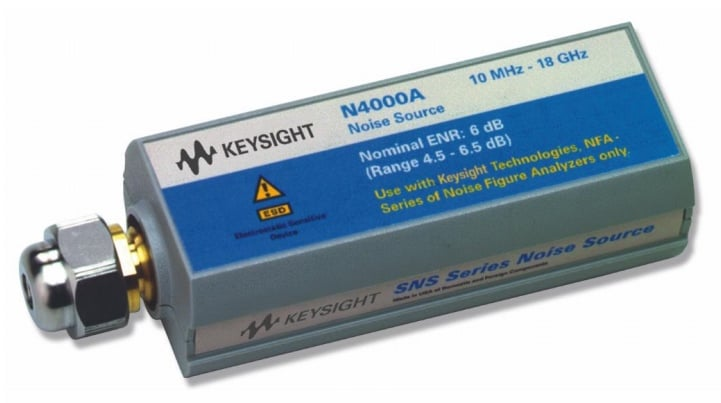##### Figure 2. The N4000A noise source. Image courtesy of Keysight Technologies

The uncertainty of the noise source ENR, supplied by the manufacturer, is one source of measurement uncertainty. The ENR uncertainty is normally in the range of 0.1 to 0.2 dB. This uncertainty contributes to the NF measurement uncertainty, often almost dB-for-dB. For example, if the ENR measurement uncertainty is 0.3 dB, the noise figure measurement uncertainty is also 0.3 dB.

Note that the ENR measurements are made at a number of discrete frequencies (e.g., at 10 or 20 frequency points) over the noise source’s operating frequency range. However, since the ENR of a well-designed noise source changes only gradually with frequency, the measurement equipment is able to interpolate the noise source performance between the calibration points without incurring a significant error.

### Selecting a Noise Source: Low vs. High ENR

The most common ENR values for commercial noise sources are 5, 6, and 15 dB. The remaining question is, what is the appropriate nominal ENR for a given measurement? Recall that ENR is a way of specifying the noise level difference between the hot and cold states of the noise source.

With a lower ENR, the measurement equipment processes a smaller noise level during the hot measurement. This has two advantages: first, the measurement equipment is more likely to remain in its linear region of operation unless the DUT has a very large gain. Second, the measurement instrument uses a smaller internal attenuation factor, and hence, it should have a smaller noise figure. Therefore, the error from the instrument’s nonlinearity and noise figure can be minimized by using a lower ENR noise source.

However, there is another factor that needs to be considered: a low ENR cannot be used when the DUT’s noise figure is high. To understand this, note that if the DUT’s noise figure is too large relative to the ENR of the noise source, the output noise mainly stems from the DUT itself. Therefore, the output noise during the hot and cold measurements is almost the same Nh ≅ Nc, leading to Y ≅ 1. This consequently makes the denominator of Equation 2 approach zero. In this case, a small error in Y leads to a large error in measured equivalent noise temperature Te.

It is recommended to use a noise source with ENR that is 10 dB less than the noise figure of the DUT. For example, a 6 dB ENR noise source can be used for DUTs whose noise figure doesn’t exceed about 16 dB. And, a 15 dB ENR noise source is suitable in NF measurements up to about 25 dB.

### Reducing Mismatch Uncertainty Through Attenuators

In general, impedance mismatch at different ports of an RF signal chain can cause power transfer uncertainty. This effect can be a significant error source in noise figure measurements. Attenuators can limit the mismatch uncertainty, so a natural question to ask at this point is, can we use attenuators to reduce the uncertainty of noise figure measurements?

To answer this, consider the diagram of Figure that shows the measurement step of the Y-factor method with attenuators added before and after the DUT.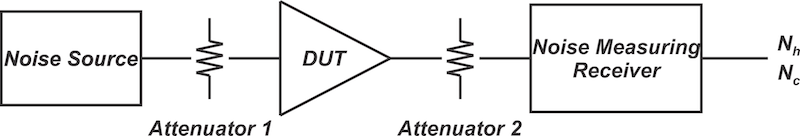##### Figure 3. Y-factor measurement configuration with attenuators added

While attenuators improve the effective return loss of a port and reduce the mismatch uncertainty, they also attenuate the desired signal and introduce noise. Therefore, without a complete analysis, it is not possible to assess if an attenuator improves the overall measurement accuracy or not.

Figure 4 shows the effect of adding attenuators on the noise figure measurement uncertainty of a DUT with NF of 3 dB for different values of DUT gain. The NI application note associated with the figure doesn’t give the specific value of the DUT’s input return loss, but it mentions that the DUT has a poor input return loss.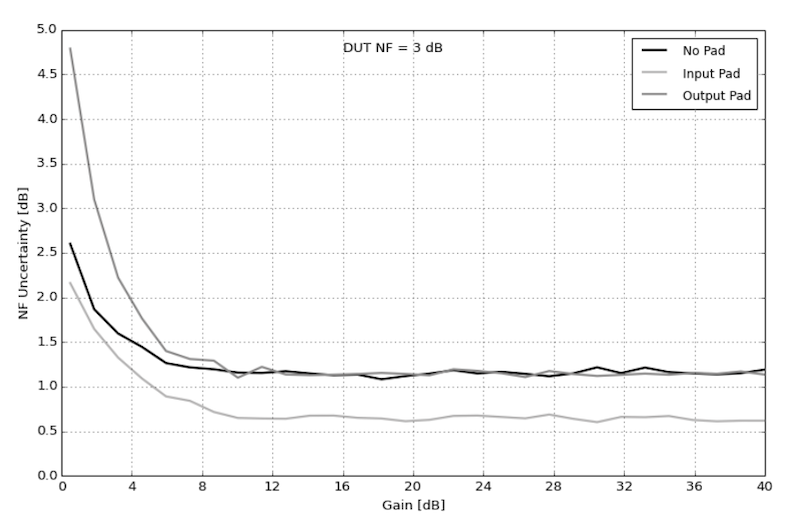##### Figure 4. NF uncertainty as a function of gain. Image used courtesy of National Instruments

As expected, by adding the input pad, the impedance mismatch and the measurement uncertainty are reduced. However, adding the output pad doesn’t reduce the measurement uncertainty in this example. This is due to the fact that adding the output attenuator degrades the effective noise figure of the “noise measuring receiver”. Let’s see how the loss of the attenuators can be accounted for.

### Losses Before the DUT

To have accurate noise figure measurements, it is necessary to correct for any loss that precedes the DUT. One source of pre-DUT losses is the cable attenuation. Also, sometimes the noise source connector is not compatible with the DUT, and an adapter is needed to insert the DUT into the measurement loop. The loss of the adapter can also affect our measurement.

During the calibration step, the noise source is directly connected to the measurement equipment. This automatically establishes the measurement reference plane at the output of the noise source (Figure 5). The calibration step determines the noise temperature of the receiver TReceiver.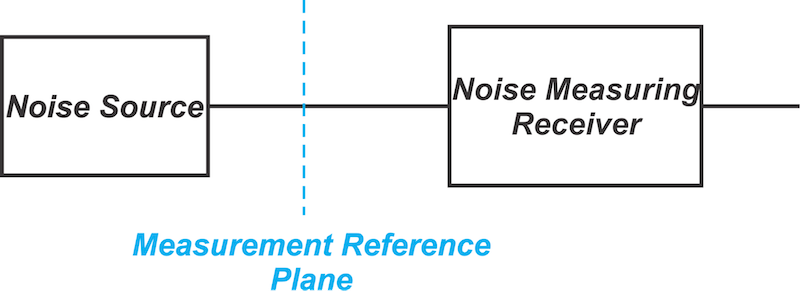##### Figure 5. The calibration step of the Y-factor method.

The block diagram of the measurement step with a pre-DUT attenuator is shown in Figure 6.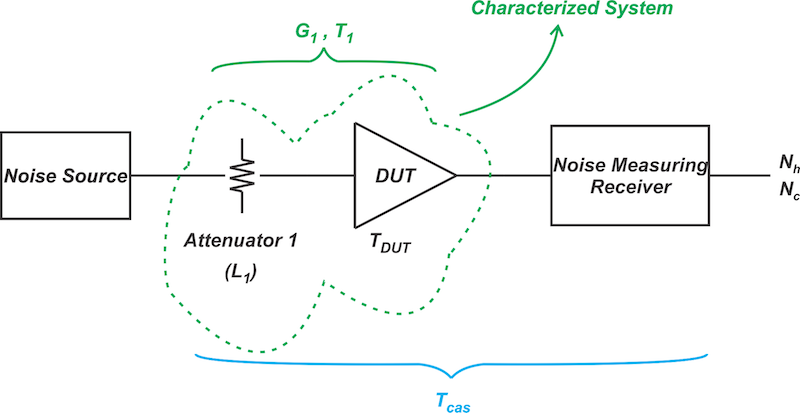##### Figure 6. The measurement step of the Y-factor method.

With the above diagram, the two-stage cascade of the attenuator and the DUT are characterized. Assume that the measurement finds the noise temperature of this two-stage system to be T1. Applying Friis’s equation, we have:

$$T_1 = T_{atten} + \frac{T_{DUT}}{G_{atten}}$$

##### Equation 3

In Equation 3, Tatten and Gatten are the noise temperature and gain of the attenuator. The gain of a lossy network is less than unity.

For an attenuator, we can define the loss factor as the reciprocal of its gain, i.e. L1 = 1/Gatten. Here, L1 is in linear terms rather than decibels and has a value greater than unity. Also, the noise temperature of an attenuator with a loss factor of L1 at physical temperature TL is given by Tatten = (L1-1)TL. Hence, we have:

$$T_1 = (L_1 - 1)T_L + T_{DUT}L_1$$

##### Equation 4

We can easily express the above equation in terms of the noise factor values:

$$F_1 = F_{atten} + (F_{DUT} - 1)L_1$$

##### Equation 5

It is instructive to consider the special case where the attenuator is at the standard temperature T0 = 290 K. Let’s look at an example.

#### Example: The Special Case of TL = T0

Assume that the pre-DUT attenuator is at physical temperature T0 and has a loss of L1 = 1.5 (or Gatten = 0.667). If the noise figure meter measures a composite noise figure of 7 dB, what is the DUT’s actual noise figure?

We discussed in a previous article that when the physical temperature of a passive attenuator with a loss of L is T0 = 290 K, then its noise factor is F = L (or equivalently, its noise figure in dB is equal to its loss in dB). Substituting Fatten = L1 into Equation 5 produces:

$$F_1 = L_1 + (F_{DUT} - 1)L_1 = F_{DUT}L_1$$

##### Equation 6

Or equivalently:

$$NF_1 = NF_{DUT} + NF_{atten}$$

##### Equation 7

The noise figure of an attenuator with L1 = 1.5 is NFatten = 1.76 dB. Therefore, we have:

$NF_{DUT} = 7 - 1.76 = 5.24 \text{ dB}$

### Potential Noise Figure Errors

In the above analysis, NFatten is subtracted from the composite noise figure NF1 to determine the DUT’s noise figure. This procedure can lead to a significant error if NFatten is comparable with NFDUT, and NFatten has a relatively large measurement uncertainty.

For example, assume that NFDUT and NF1 are 2 dB and 4 dB, respectively. The actual value of NFatten is also 2 dB; however, due to measurement uncertainties, we have measured it to be 2.5 dB. Based on this measured value, the DUT’s noise figure is NFDUT = 4 - 2.5 = 1.5 dB, which corresponds to a measurement error of 25%.

It’s also worthwhile to mention that the loss term might be frequency dependent. In this case, the loss data at the frequency of interest should be used to correct for the loss effect.

### Losses After the DUT

Since the calibration step establishes the measurement reference plane at the output of the noise source (Figure 5), we can include the components that follow the DUT in the calibration loop to account for their losses. This automatically absorbs all the losses that appear after the DUT into the measurement equipment’s noise figure. This is illustrated below in Figure 7.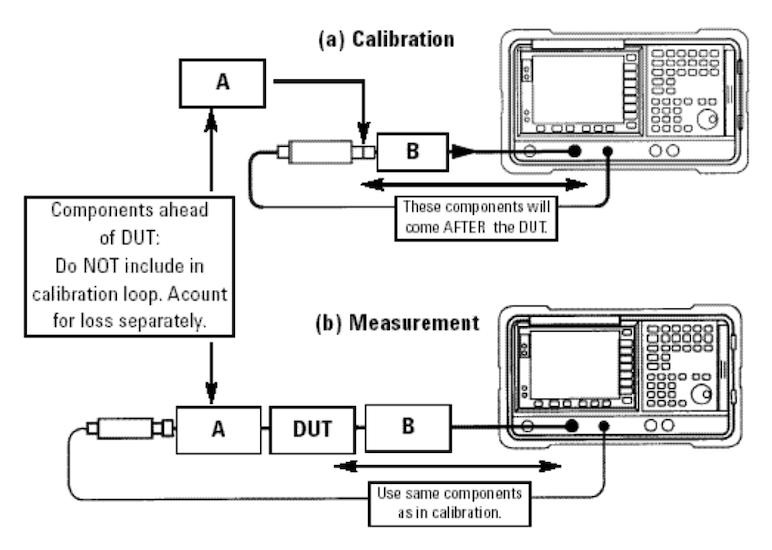##### Figure 7. Noise figure test configuration to account for losses after the DUT. Image used courtesy of Agilent Technologies

In Figure 7, component B, which comes after the DUT, is also included in the calibration step. However, component A cannot be included in the calibration step, and its loss should be accounted for separately, as explained in the previous section.

If a loss that comes after the DUT cannot be included in the calibration loop, then we’ll have to correct the measurement results for that particular loss. Consider the block diagram of Figure 8, which demonstrates a measurement step where a lossy component is between the DUT and the measuring equipment. In this figure, we’re assuming that it was impossible to include this lossy component in the calibration loop.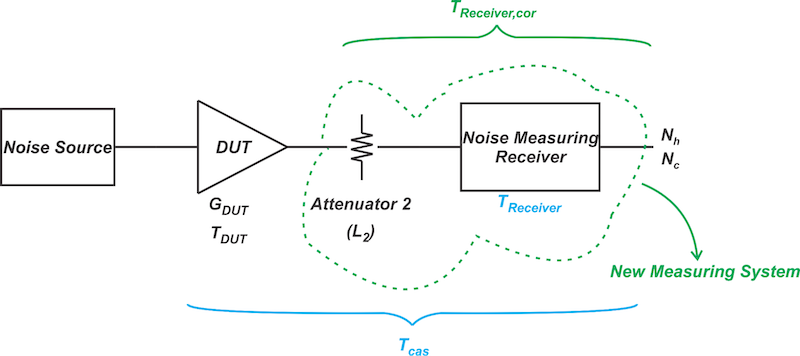##### Figure 8. NF measurement with a loss component between the DUT and the measurement receiver

Using the above diagram, we can derive an equation that absorbs the effect of the attenuator into the measuring equipment’s noise figure. Applying Friis’s equation, the corrected noise temperature for the measuring instrument is:

$$T_{receiver,cor} = T_{atten} + \frac{T_{receiver}}{G_{atten}}$$

##### Equation 9

As discussed in the previous section, we have Gatten = 1/L2 and Tatten= (L2-1) TL where TL is the physical temperature of the attenuator. Substituting these values yields the corrected noise temperature for the measurement equipment:

$$T_{receiver,cor} = (L_2 - L_1)T_L + T_{receiver}L_2$$

##### Equation 10

Note that TReceiver is obtained from the calibration step. The measurement step also yields the composite gain of the DUT and the attenuator G1 = GDUT/L2. Therefore, the DUT gain is GDUT = G1L2.

Now, we can define Tcas to correspond to the whole cascade system, including the DUT, the attenuator, and the measuring equipment. We can find the DUT's noise temperature with Tcas, TReciever,cor, and GDUT.

### For Losses Before and After the DUT

As a final note, if your measurement has losses both before and after the DUT, you’ll need to use a somewhat more complicated analysis to correct for the loss errors. For more information, refer to the Keysight application note I mentioned above.

Hopefully, this article has provided you with some additional insights into noise figure measurements and the application of the Y-factor method. Using variable attenuators instead of a calibrated power meter can reduce test equipment costs. Whatever noise power measurement you employ, it is always important to consider noise source uncertainty and fixturing losses that add errors to your noise figure measurements.